## ISC Computer Science Previous Year Question Paper 2015 Solved for Class 12

Maximum Marks: 70
Time allowed: 3 hours

• Candidates are allowed additional. 15 minutes for only reading the paper.
• They must NOT start writing during this time.
• Answer all questions in Part-I (compulsory) and seven questions from Part – II, choosing three questions from Section-A, two from Section-B and two from Section-C.
• All working, including rough work, should be done on the same sheet as the rest of the answer.
• The intended marks for questions or parts of questions are given in brackets [ ].

Part – I

While answering questions in this Part, indicate briefly your working and reasoning, wherever required.

Question 1.
(a) Simplify: (A + C). (A + A.D) + A.C + C 
(b) Draw a logic circuit for (A + B).(C + D). C 
(c) Verify the following proposition with the help of a truth table: 
P ∨ (~P ∧ Q) = P ∨ Q
(d) State De Morgan’s law and verify it, using a truth table. 
(e) Answer the questions related to the circuit given below:(i) Give the output if, X = 1 and Y = 0
(ii) Name the basic gate represented by the above diagram.
(a) (A + C). (A + A.D) + A.C + C
= (A + C).(1 + D).A + C(1 + A) [∵ 1 + D = 1]
= (A + C).A + C [∵ 1 + A = 1]
= A.A + A.C + C [∵ A.A = A]
= A + C(A + 1)
= A + C [∵ A + 1 = 1]

(b) Logic circuit for (A + B). (C + D). C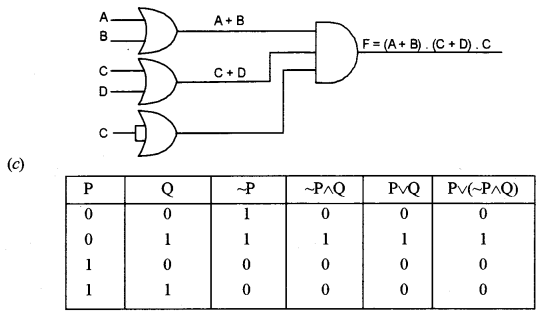(d) The two theorems suggested by De Morgan which are extremely useful in Boolean Algebra are as following:
Theorem 1
$$\overline{\mathrm{A} \cdot \mathrm{B}}=\overline{\mathrm{A}}+\overline{\mathrm{B}}$$
NAND = Bubbled OR
Table showing verification of the De Morgans’s first theorem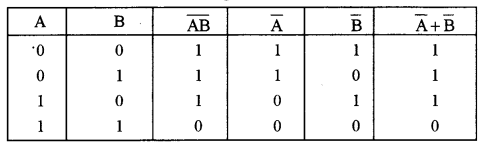Theorem 2
$$\overline{\mathrm{A}+\mathrm{B}}=\overline{\mathrm{A}} \cdot \overline{\mathrm{B}}$$
NOR = Bubbled AND
Table showing verification of the De Morgans’s second theorem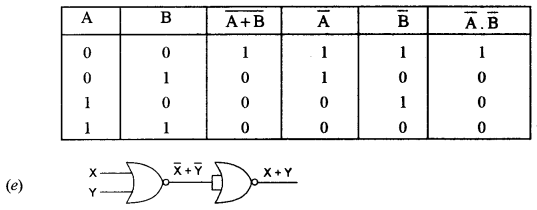(i) when X = 1 and Y = 0
the output will be 0.
(ii) NOR gate

Question 2.
(a) Define computational complexity. Calculate the complexity using Big ‘O’ notation for the following code segment: 
for(int k=0; k < n; k++)
s+ = k;
(b) Convert the following infix notation into postfix form: 
X + (Y – Z) + ((W+E)*F)/J
(c) Differentiate between this keyword and the super keyword. 
(d) The array D [-2…10][3…8] contains double type elements. If the base address is 4110, find the address of D  , when the array is stored in Column Major Wise. 
(e) State any two characteristics of a Binary tree. 
(a) Computational complexity theory focuses on classifying computational problems according to their difficulty. A problem is considered difficult if large amounts of resources (time, storage, communications, circuit gates, processors, etc.) are required to solve it, regardless of the algorithm used. A subset of computational complexity theory, known as analysis of algorithms, provides theoretical estimates for the resources needed for specific algorithms. These estimates are frequently expressed in Big O (or Big-Oh) notation, written as functions of the size of the input passed to the algorithm. For example, binary search is estimated to require a number of steps proportional to the logarithm of the length of the list being searched. In Big O notation, the estimate is written as 0(log (n)), where n = length of the list. O(N)
O(N) describes an algorithm whose performance will grow linearly and in direct proportion to the size of the input data set.

(b) = X + (Y – Z) + ((W + E) * F)/J
= X + (YZ-) + ((WE+) * F)/J
= X + (YZ-) + ((WE + F*))/J
= X + (YZ-) + (WE + F*)/J
= X + (YZ-) + (WEF*+)/J
= X + (YZ -) + (WEF * + J/)
= XYZ- + WEF* + J/+

(c) This refers to an object, that too, a current object or current class whereas super refers directly to its immediate above superclass. private variables cannot be accessed in an inherited class by using this, whereas by using “super” we can easily access the private variables in its superclass.

(d) D [-2… 10] [3 …8]
I = 4, J = 5
D   = ?
W = 8 bytes
M = 10
Columnwise
D[I, J] = B + ((J – 1) * M + (I – 1)) * W
D   = 4110 + ((5 – 1) + 10 + (4 – 1)) * 8
= 4110+ (40 + 3) × 8
= 4110 + 344
= 4454

(e) A binary tree is an important type of structure which occurs very often.

• It is characterized by the fact that any node can have at most two branches, i. e., there is no node with degree greater than two.
• For binary trees, we distinguish between the subtree on the left and on the right.
• A binary tree may have zero nodes.

Question 3.
(a) The following function is a part of some class. Assume ‘x’ and ‘y’ are positive integers, greater than 0. Answer the given questions along with dry run/working.

void someFun(int x, int y) {
if(x>1)
{
if(x%y == 0)
{
System.out.print(y+ "");
someFun(x/y, y);
}
else
someFun(x, y+1);
}
}

(i) What will be returned by someFun(24, 2)? 
(ii) What will be returned by someFun(84, 2)? 
(iii) State in one line what does the function someFun() do, apart from recursion? 
(b) The following is a function of some class which checks if a positive integer is an Armstrong number by returning true or false. (A number is said to be Armstrong of the sum of the cubes of all its digits is equal to the original number.) The function does not use modulus (%) operator to extract digit. There are some places in the code marked by ?1?, ?2?, ?3?, ?4?, ?5? which may be replaced by a statement/expression so that the function works properly.

boolean ArmstrongNum(int N)
{
int sum = ?1?;
int num= N;
while(num>0)
{
int f=num/10;
int s= ?2?;
int digit = num-s;
sum+=?3?;
num= ?4?;
}
if(?5?)
return true;
else
return false;
}

(i) What is the statement or expression at ?1? 
(ii) What is the statement or expression at ?2? 
(iii) What is the statement or expression at ?3? 
(iv) What is the statement or expression at ?4? 
(v) What is the statement or expression at ?5? 
(a) (i) 2 2 2 3 24, 2
(ii) 2 2 3 7 84, 2
(iii) someFunOcalculates the L.C.M (Lowest Common Multiple).
(b) (i) 0
(ii) 0
(iii) (f * f * f);
(iv) num/10;
(v) s == num

Part – II

Answer seven questions in this part, choosing three questions from Section-A, two from Section-B and two from Section-C

Section – A

Question 4.
(a) Given the Boolean function F (A, B, C, D) = Π (0, 1, 2, 3, 5, 7, 8, 9, 10, 11).
(i) Reduce the above expression by using 4-variable Karnaugh map, showing the various groups (i.e. octal, quads and pairs). 
(ii) Draw the logic gate diagram for the reduced expression. Assume that the variables and their complements are available as inputs. 
(b) Given the Boolean function:
P (A, B, C, D) = ABC’D’ + A’BC’D’ + ABC’D + ABC’D + ABCD + ABCD
(i) Reduce the above expression by using 4-variable Karnaugh map, showing the various groups (i.e., octal, quads and pairs). 
(ii) Draw the logic gate diagram for the reduced expression. Assume that the variables and their complements are available as inputs. 
(a) (i) F (A, B, C, D) = Π (0, 1, 2, 3, 5, 7, 8, 9, 10, 11)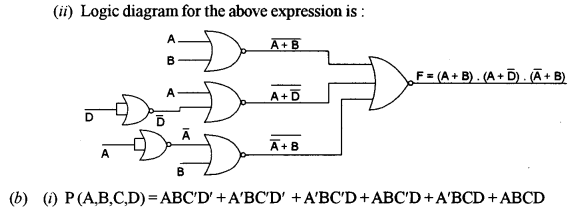Question 5.
A person is allowed to travel in a reserved coach of the train if he/she satisfies the criteria given below:
The person has a valid reservation ticket and a valid ID proof.
OR
The person does not have a valid reservation ticket but holds a valid pass issued by the Railway department with a valid ID proof.
OR
The person is a disabled person and holds a valid pass issued by the Railway department along with a valid ID proof.
The inputs are:

 INPUTS R The person has a valid reservation ticket. P The person holds a valid pass issued by the Railway department. D The person has valid ID proof. H A person is a disabled person.

(In all the above cases, 1 indicates yes and 0 indicates no).
Output: T – Denotes allowed to travel (1 indicates yes and 0 indicates no in all the cases)
(a) Draw the truth table for the inputs and outputs given above and write the POS expression for T(R, P, D, H). 
(b) Reduce T(R, P, D, H) using the Karnaugh map.
Draw the logic gate diagram for the reduced POS expression for T (R, P, D, H) using only NOR gates. You may use gates with two or more inputs, assume that the variable and their complements are available as inputs. 
(a) Truth Table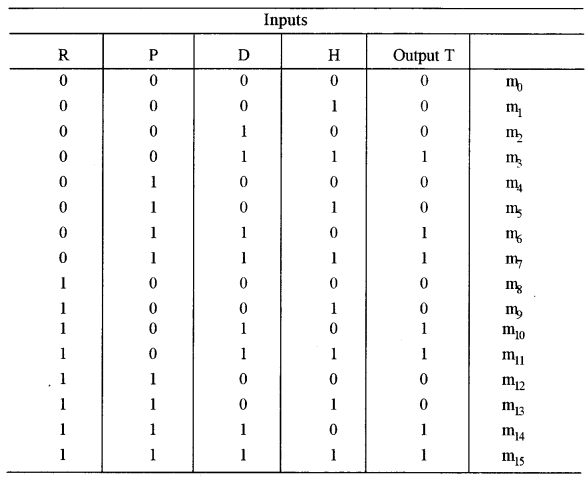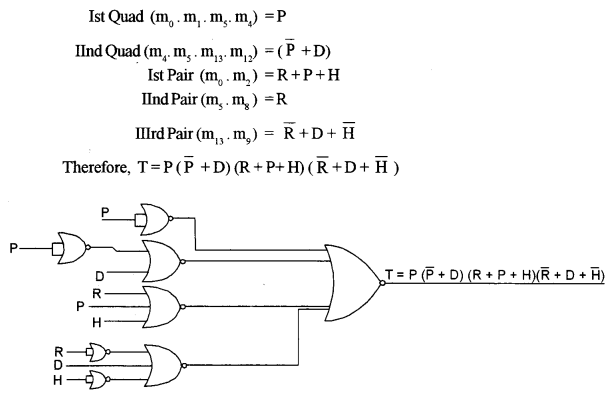Question 6.
(a) Draw the truth table and logic gate diagram for an Octal to Binary encoder. 
(b) What is Multiplexer? State an application of a Multiplexer. Also, draw the logic diagram of a 4 : 1 Multiplexer. 
(c) Verify the following expression using Boolean laws. Also, mention the law used at each step of simplification. 
X.Y.Z + X.Y’.Z + X.Y.Z = X.(Y + Z)
(a) An encoder has 2n or fewer input lines, only one of which is in the “1” state at a particular time and an n-bit code is generated on “n” output lines depending upon which of the input is excited. In other words, an encoder is a circuit in which output lines generate the binary code corresponding to the input value.Octal To Binary Encoder
Octal to binary encoder has 23 = 8 input lines D0 to D7 and 3 output lines Y0 to Y2. Below is the truth table for octal to the binary encoder.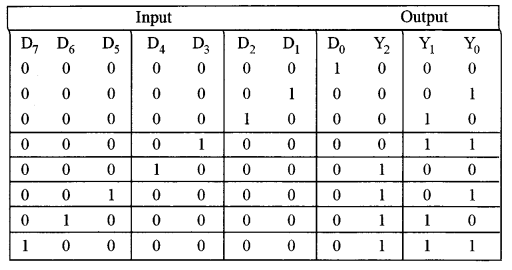From the truth table, the outputs can be expressed by following Boolean Function.
Y0 = D1 + D3 + D5 + D7
Y1 = D2 + D3 + D6 + D7
Y2 = D4 + D5 + D6 + D7
Note: Above boolean functions are formed by OR ing all the input lines for which output is 1. For instance Y0 is 1 for D1, D3, D5, D7 input lines.
The encoder can, therefore, be implemented with OR gates whose inputs are determined directly from the truth table as shown in the image below: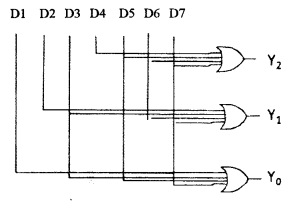(b) The Multiplexer acts as a multiple-input and single-output switch.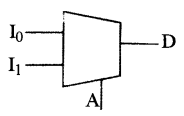Multiplexers switch two or more input signals to an output port. This allows multiple information streams to be sent over one transmission channel. Since the multiplexer spends some time on each input, the time is divided between the inputs.
Applications are cellphone systems, instrumentation, and any other function where only one transmission channel (e.g., a radio transmitter) is available, yet information from several sources must be sent.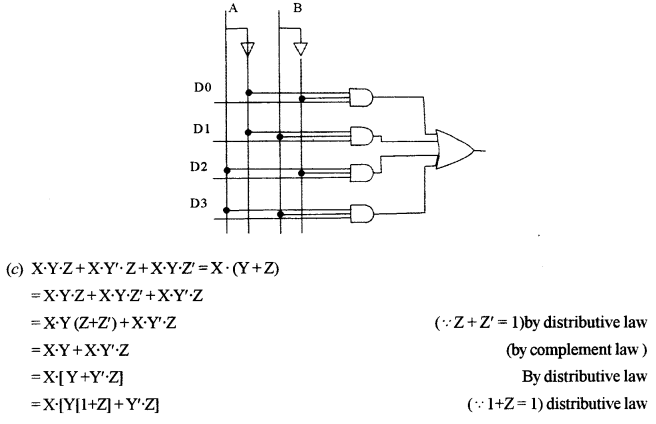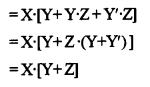Question 7.
(a) Derive a Boolean expression for the logic circuit given below and reduce the derived expression, using Boolean laws: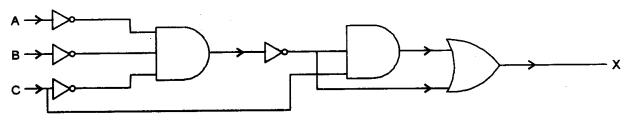(b) What are the universal gates? Construct a logic circuit using NAND gates only for the expression: 
(c) Define Half Adders. Draw the circuit diagram and the truth table for a Half Adder.Boolean Expression for the above circuit is
A.B.C.C + A.B.C
To simplify this expression,
F = A.B.C.C + A.B.C
= A.B.C + A.B.C (∵C.C = C)
= A.B.C [∵a + a = a]
(b) NOR and NAND gates have the particular property that any one of them can create any logical Boolean expression if designed in a proper way.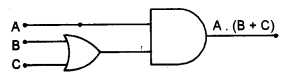(c) The half adder is an example of a simple, functional digital circuit built from two logic gates. The half adder adds to one-bit binary numbers (AB). The output is the sum of the two bits (S) and the cany (C)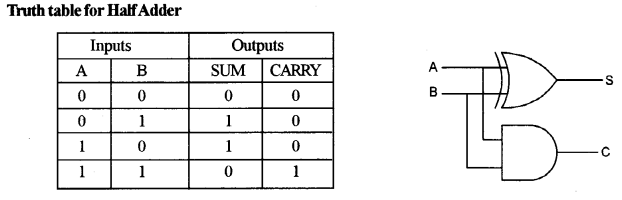Section – B

• Each program should be written in such a way that it clearly depicts the logic of the problem.
• This can be achieved by using mnemonic names and comments in the program.
• Flowcharts and algorithms are not required.
• The programs must be written in Java.

Question 8.
A class Admission contains the admission numbers of 100 students. Some of the data members/member functions are given below: 
Data member/instance variable:
Member functions/methods:
Admission(): constructor to initialize the array elements
void fill Array(): to accept the elements of the array in ascending order
int binSearch(int 1, int u, int v): to search for a particular admission number (v) using binary search and recursive technique and returns 1 if found otherwise returns -1
Specify the class Admission giving details of the constructor, void fill Array() and int binSearch(int, int, int). Define the main() function to create an object and call the functions accordingly to enable the task.

import java.util. Scanner;
{
}
public void fillArray()
{
Scanner sc=new Scanner(System.in);
System.out.print(“Enter admission numbers in ascending order:”);
{
}
}
public int binSearch(int 1, int u, int v)
{
int m=(1+u)/2;
if(1>u)
return-1;
return 1;
return binSearch(m+1, u, v);
else
return binSearch(1, m-1, v);
}
public static void main(String args[])
{
Scanner sc = new Scanner(System.in);
obj.fillArray();
System.out.print("Enter the key to search:");
int key = sc.nextInt();
int result = obj .binSearch(0, 4, key);
if(result == 1)
System.out.println(key+"available.");
else
System.out.println(key +" unavailable.");
}
}

Question 9.
A class Merger concatenates two positive integers that are greater than 0 and produces a newly merged integer. 
Example: If the first number is 23 and the second is 764, then the concatenated number will be 23764.
Some of the members of the class are given below:
Class name: Merger
Data members/instance variables:
n1: long integer to store the first number
n2: long integer to store the second number
mergNum: long integer to store the merged number
Member functions:
Merger(): constructor to initialize the data members
void readNum(): to accept the values of the data members n1 and n2
voidjoinNum(): to concatenate the numbers n1 and n2 and store it in
mergNum
void show(): to display the original numbers and the merged number with appropriate messages
Specify the class Merger giving the details of the constructor, void readNum(), void joinNum() and void show(). Define the main() function to create an object and call the functions accordingly to enable the task.

(a) import java util. Scanner;
class Merger
{
long n1; long n2;
long mergNum;
public Merger()
{
n1=1;
n2=1;
mergNum=11;
}
{
Scanner sc=new Scanner(System.in);
System.out.print("Enter first number:");
n1=(int)Math.abs(sc.nextLong());
System.out.print("Enter second number:");
n2=(int)Math.abs(sc.nextLong());
if(n1==0)
n1=1;
if(n2==0)
n2=1;
}
public void joinNum()
{
String num1 = Long.toString(n1);
String num2 = Long.toString(n2);
String merged=num1 + num2;
mergNum=Long.parseLong(merged);
}
public void show()
{
System.out.println("First Number: "+n1):
System.out.println("Second Number: "+n2):
System.out.println("Merged Number: "+mergNum):
}
public static void main(String args[])
{
Merger obj=new Merger));
Obj.joinNum();
obj.show));
}
}

Question 10.
A class TheString accepts a string of a maximum of 100 characters with only one blank space between the words. 
Some of the members of the class are as follows:
Class name: TheString
Data members/instance variables:
str: to store a string
len: integer to store the length of the string
wordCount: integer to store the number of words
cons: integer to store the number of consonants
Member functions/methods:
TheString(): default constructor to initialize the data members
TheString(String ds): parameterized constructor to assign str=ds
void countFreq(): to count the number of words and the number of consonants and store them in wordCount and cons respectively
void display(): to display the original string, along with the number of words and the number of consonants
Specify the class TheString giving the details of the constructors, void countFreq() and void display(). Define the main() function to create an object and call the functions accordingly to enable the task.

import java.util. Scanner;
class TheString
{
String str;
int len;
int wordCount;
int cons;
public TheString()
{
str=new String();
len=0;
wordCount=0;
cons=0;
}
public TheString(String ds)
{
str=ds;
if(str. length()> 100)
str=str. substring(0, 101);
len=str.length();
wordCount=0;
cons=0;
}
public void countFreq()
{
int i;
for(i=0;i<len;i++)
{
char ch=str.charAt(i);
if(ch=")
wordCount++;
ch=Character.toUpperCase(ch);
switch(ch)
{
case 'A':
case'E':
case 'I':
case 'O':
case 'U':
break;
default:
ij(ch>='A' && ch<='Z')
cons++;
}
}
if(len>0)
wordCount++;
}
public void display()
{
System. out.println("Original String :"+str);
System.out.println("Number of words: "+wordCount);
System.out.println("Number of consonants:"+cons);
}
public static void main(String args[])
{
Scanner sc=new Scanner(System.in);
System.out.print("Enter the string:");
String s=sc.nextLine();
TheString obj=new TheString(s);
obj.countFreq();
obj.display();
}
}

Section – C

• Each program should be written in such a way that it clearly depicts the logic of the problem stepwise.
• This can be achieved by using comments in the program and mnemonic names or pseudo-codes for algorithms.
• The programs must be written in Java and the algorithms must be written in general/standard form, wherever required/specified.
• Flowcharts are not required.

Question 11.
WordPile is an entity which can hold a maximum of 20 characters. The restriction is that a character can be added or removed from one end only.
Some of the members of classes are given below:
Class name: WordPile
Data members/instance variables:
ch[]: character array to hold the character elements
capacity: integer variable to store the maximum capacity
top: to point to the index of the topmost element
Member functions/methods:
WordPile (int cap): constructor to initialise the data member
capacity = cap, top = -1 and create the WordPile
void pushChar(char v): adds the character to the top of WordPile if possible, otherwise output a message “WordPile is full”
charpopChar(): returns the deleted character from the top of the WordPile if possible, otherwise it returns ‘\\’
(a) Specify the class WordPile giving the details of the constructor, void pushChar(char) and char popChar(). 
The main function and algorithm need not be written.
(b) What is the name of the entity described above and state one of its applications? 

(a) import java. util. Scanner;
class WordPile{
char ch[];
int capacity;
int top;
public WordPile(int cap)
{
capacity=cap;
top=-1;
ch=new char[capacityj;
}
public void pushChar(char v)
{
if(top+1==capacity)
System.out.println("WordPile is full");
else
ch[++top]=v;
}
public char popChar()
{
if(top==-1)
return '\\';
else
return ch[top--];
}
//main written so that the program can be executed
public static void main(String args[]){
Scanner sc=new Scanner(System.in);
System.out.print("How many characters?”);
int n=sc.nextInt();
if(n>20)
n=20;
WordPile obj=new WordPile(n);
outer:
while(true)
{
System.out.println("1. PushCharacter");
System.out.println("2. Pop Character");
int choice=sc.nextInt();
switch(choice)
{
case 1:
System.out.print("Enter thecharacter:");
char c=sc.next()charAt(0);
obj.pushChar(c);
break;
case 2:
c=obj.popChar();
if(c=='\\')
System.out.println("Empty stack.");
else
System.out.println(c+" popped.");
break;
default:
break outer;
}
}
}
}

(b) The name of the entity described above is ‘Stack’. One of its applications is to reverse a string.

Question 12.
A line on a plane can be represented by coordinates of the two-end points p1 and p2 as p1(x1, y1) and p2(x2, y2).
A superclass Plane is defined to represent a line and a subclass Circle to find the length of the radius and the area of the circle by using the required data members of the superclass. 
Some of the members of both classes are given below:
Class name: Plane
Data members/instance variables:
x1: to store the x-coordinate of the first endpoint
y1: to store the y-coordinate of the first endpoint
Member functions/methods:
Plane (int nx, int ny): parameterized constructor to assign the data members x1 = nx and y1 = ny
void show(): to display the coordinates
Class name: Circle
Data members /instance variables:
x2: to store the x-coordinate of the second endpoint
y2: to store the y-coordinate of the second endpoint
area: double variable to store the area of the circle
Member functions/methods:
Circle(…): parameterized constructor to assign values to data members of both the classes
void findRadius(): to calculate the length of the radius using the formula:
$$(\sqrt{(x 2-x 1)^{2}+(y 2-y 1)^{2}}) / 2$$
assuming that x1, x2, y1, y2 are the coordinates of the two ends of the diameter of a circle
voidfindArea(): to find the area of a circle using the formula: πr2. The value of pie(π) is 22/7 or 3.14
void show(): to display both the coordinates along with the length of the radius and area of the circle
Specify the class Plane giving details of the constructor and void show() Using the concept of inheritance, specify the class Circle giving details of the constructor, void findRadius(), void find Area() and voidShow()
The main function and algorithm need not be written.

class Plane
{
int x1;
int y1;
public Plane(int nx, int ny)
{
x1=nx;
y1=ny;
}
public void show()
{
System.out.println("P1: "+x1 +", "+y1);
}
}
class Circle extends Plane{
int x2;
int y2;
double area;
public Circle(int nx1, int ny1, int nx2, int ny2)
{
super(nx1, nx2);
x2=nx2;
y2=ny2;
}
{
}
public void findArea()
{
}
public void show(){
super. show();
System.out.println("P2: "+x2+", "+y2);
System.out.println("Area: "+area);
}
}
class Coordinate
{
//main method created so that the program can be executed
public static void main(String args[])
{
Circle obj=new Circle(2, 3, 4, 5);
obj.findArea();
obj.show();
}
}

Question 13.
(a) A linked list is formed from the objects of the class: 

class Nodes
{
int num;
Node next;
}

Write an Algorithm OR a Method to print the sum of nodes that contains only odd integers of an existing linked list.
The method declaration is as follows:
void NodesCount (Nodes starPtr)
(b) (i) Give the meaning of the following common expression in Big O notation: 
O(N)
O(N2)
(ii) List any two cases to analyse algorithm complexities. 
(c) Answer the following from the diagram of a Binary Tree given below:(i) Name the leaf nodes of the right sub-tree. 
(ii) Write postorder traversal of the left subtree of node B including itself. 
(iii) State the level number of nodes R and M when the root is at level 0. 
(iv) Name the internal nodes of the tree. 

(a) public void nodesCount(Nodes startPtr)
{
int sum=0;
while(startPtr!=null)
{
if(startPtr.num%2=1)
sum=sum+startPtr.num;
startPtr=startPtr.next;
}
System.out.println(sum);
}

(b)
(i) O(N) is a computational complexity which is Linear in nature.
O(N2) is a computational complexity which is Quadratic in nature.
(ii) Two cases to analyze algorithm complexities: Worst Case, Best Case.
(c)
(i) Nodes P and E are the leaf nodes of the right sub-tree.
(ii) R, N, M, C, B is the required post-order traversal.
(iii) 2
(iv) C, F, M, H are the internal nodes.